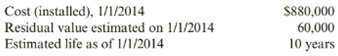Chapter 7, Problem 59E### Cornerstones of Financial Accounti...

4th Edition
Jay Rich + 1 other
ISBN: 9781337690881

#### Solutions

Chapter
Section### Cornerstones of Financial Accounti...

4th Edition
Jay Rich + 1 other
ISBN: 9781337690881
Textbook Problem
300 views

# Disposal of Fixed AssetPacifica Manufacturing retired a computerized metal stamping machine on December 31, 2019. Pacifica sold the machine to another company and did not replace it. The following data are available for the machine:The machine was sold for \$225,000 cash. Pacifica uses the straight-line method of depreciation.Required:1. Prepare the journal entry to record depreciation expense for 2019.2. Compute accumulated depreciation at December 31, 2019.3. Prepare the journal entry to record the sale of the machine.4. CONCEPTUAL CONNECTION Explain how the disposal of the fixed asset would affect the 2019 financial statements. Ignore income taxes.

To determine

Concept introduction:

Straight-line Depreciation:

Depreciation is the process in which the cost of the fixed assets other than land is allocated to expense over the useful life of the asset. The most commonly used method of depreciation is straight line method. In this method every year until the asset’s useful life an equal amount of an assets cost to depreciation expense is allocated. The formula to calculate straight line depreciation is:

Straight line depreciation = (cost − residual value) / expected useful life

Requirement 1:

Prepare journal entry to record depreciation expense for 2019.

Explanation

First we have to determine the depreciation expense for 2019 and to calculate the expense let’s use the following formula:

Straight line depreciation = (cost − residual value) / expected useful li...

To determine

Concept introduction:

Straight-line Depreciation:

Depreciation is the process in which the cost of the fixed assets other than land is allocated to expense over the useful life of the asset. The most commonly used method of depreciation is straight line method. In this method every year until the asset’s useful life an equal amount of an assets cost to depreciation expense is allocated. The formula to calculate straight line depreciation is:

Straight line depreciation = (cost − residual value) / expected useful life

Requirement 2:

To explain:

Compute accumulated depreciation at Dec 31 2019.

To determine

Concept introduction:

Disposal of fixed assets:

Fixed assets are disposed by a company either voluntarily or involuntarily. Voluntary disposal occurs when the useful life of the asset is over or due to technological obsolescence. Involuntary disposal occurs due to damage from fire, theft or natural calamities.

Requirement 3:

To explain:

Prepare the journal entry to record the sale of the machine.

### Still sussing out bartleby?

Check out a sample textbook solution.

See a sample solution

#### The Solution to Your Study Problems

Bartleby provides explanations to thousands of textbook problems written by our experts, many with advanced degrees!

Get Started

#### Find more solutions based on key concepts